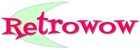# Convert pounds and ounces to kg

Pounds (lb)

Ounces (oz)

Kilograms (kg) kg

Grams (g) g

Total weight in grams g

In the imperial system of measurement one pound is sixteen ounces. In the metric system 1000 grams are one kilogram. This page converts the imperial weight in pounds and ounces to the metric weight in kilograms and grams.

1 pound (1 lb) = 0.454 kg or 454g

1 ounce (1 oz) = 28.3g